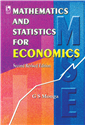Home > Higher Education > Commerce And Management > Mathematics and Statistics for Economics# Mathematics and Statistics for Economics, 2/e

## G.S. Monga

 ISBN : 9788125909828 Pages : 912 Binding : Paperback Language : English Imprint : Vikas Publishing Trim size : 6.25 X 9.5 inches Weight : 0.50kg © year : 2000
 List Price :650.00
 Offer Price :520.00

20% OFF

# In Stock

Credit Card / Debit Card / Internet BankingThe purpose of the book is to provide technique of salient mathematical and statistical methods widely used by economists and managers in various contexts. The contents and methodology of this book have been tested over the past three decades and more. Each chapter gives a concise presentation of concepts and theories which are illustrated and simplified with the help of examples and solved problems. As the book is intended for the beginners, although a wide range of topics have been covered the treatment is kept at the elementary level. The students will easily engage with the subject once they start reading the chapters and practice the unsolved questions. • Has a very wide coverage (30 chapters), so that the book can be used in a wide range of courses. • Includes 100s of solved and unsolved numerical problems. Final answers to the unsolved questions have been given. Hints to difficult problems are given. • Both solved and unsolved examples are given at the topic level rather than the chapter level, so that the students progress to the next topic after understanding the previous one. 1. Review of Some Elementary Mathematics, 2. Mathematical Reasoning, 3. Sets and Relations, 4. The Number System, 5. System of Equations and Inequations Market Equilibrium, 6. Trigonometry, 7. Determinants and Matrices, 8. Analytical Geometry, 9. Functions and Limits, 10. Differential Calculus: Functions of One Variable, 11. Differential Calculus: Functions of Two or More Variables, 12. Integral Calculus, 13. Differential and Difference Equations, 14. Input Output Economics, 15. National Income and Economic Accounting, 16. Linear Programming, 17. Probability and Statistics, 18. Frequency Distributions, 19. Measures Central Tendency, 20. Dispersion, Descriptive Measures, Expectation, Movements, 21. Distributions-I: Some Theoretical Distributions, 22. Distribution-II: Discrete and Continuous Distributions Exact Sampling Distributions, 23. Correlation and Regression, 24. Sampling, 25. Statistical Inference: Estimation, 26. Testing of Hypotheses, 27. Analysis of Variance (Anova), 28. Index Numbers, 29. Time Series, 30. Compilation and Presentation of Statistics650.00520.00